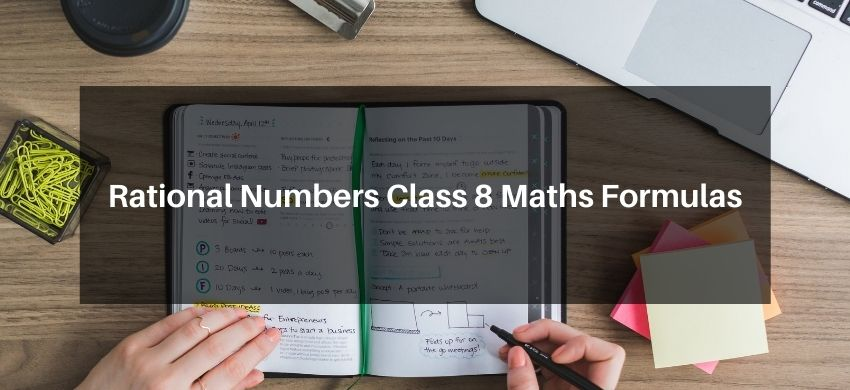# Rational Numbers Class 8 Maths FormulasHey, students are you looking for Rational Numbers Class 8 Maths Formulas? If yes. Then you are at the right place. In this post, I have listed all the formulas of Rational Numbers class 8 that you can use to learn and understand the concepts easily.

If you want to improve your class 8 Math, Rational Number concepts, then it is super important for you to learn and understand all the formulas.

By using these formulas you will learn about Rational Numbers.

With the help of these formulas, you can revise the entire chapter easily.

## Rational Numbers Class 8 Maths Formulas

• Rational Number: A number is called rational if we can write the number in the form of $\frac{p}{q}$, where $p$ and $q$ are integers and $q \neq 0$ i.e., $1=\frac{1}{1}, 2=\frac{2}{1}, 0=\frac{0}{1}$ and $\frac{5}{8}, \frac{-3}{14}, \frac{7}{-15}$ are all rational numbers.
• Additive Identity: $(a / b+0)=(a / b)$
• Multiplicative Identity: $(\mathrm{a} / \mathrm{b}) \times 1=(\mathrm{a} / \mathrm{b})$
• Closure Property - Addition: For any two rational numbers a and $b, a+b$ is also a rational number.
• Closure Property - Subtraction: For any two rational numbers a and $b, a-$ $b$ is also a rational number.
• Closure Property - Multiplication: For any two rational numbers a and $b, a x$ $b$ is also a rational number.
• Closure Property - Division: Rational numbers are not closed under division.
• Commutative Property - Addition: For any rational numbers a and $b, a+b=$ $b+a$.
• Commutative Property - Subtraction: For any rational numbers a and b, a $b \neq b-a$.
• Commutative Property - Multiplication: For any rational numbers $a$ and $b$, $(a x b)=(b x a)$.
• Commutative Property - Division: For any rational numbers a and $b,(a / b) \neq$ (b/a).
• Associative Property - Addition: For any rational numbers $a, b$, and $c,(a+$ $b)+c=a+(b+c)$.
• Associative Property - Subtraction: For any rational numbers $a, b$, and c, $(a$ $-b)-c \neq a-(b-c)$
• Associative Property - Multiplication: For any rational number $a, b$, and c, $(a \times b) \times c=a \times(b \times c)$.
• Associative Property - Division: For any rational numbers $a, b$, and $c$, ( $a$ /b) / $\mathrm{c} \neq \mathrm{a} /(\mathrm{b} / \mathrm{c})$.
• Distributive Property: For any three rational numbers $a, b$ and $c, a \times(b+c)$ $=(a \times b)+(a \times c)$.